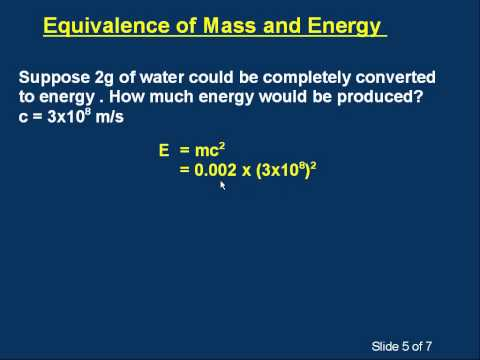# Explaining E=mc^2?

Relevance
• That is just a result of calculations involving relativistic transforms ...

That is the rest mass energy formula it basically is the energy needed to push the particles together to makes the object

•Log in to reply to the answers
• Energy can be created from matter. Energy equals mass times speed of light squared.

•Log in to reply to the answers
• During a fission nuclear reaction, nucleus of a radioactive substance splits into two less reactive substances. One example is the fission of Uranium. The products can be Zenon and Barium and a very large of energy. We know the mass of one mole of each of these elements. An amazing discovery is that the sum of the mass of the Xenon and the Barium is less than the mass of the Uranium. The equation below determines the relationship between the decease of the mass and the amount of energy that produced

E = m * c^2

The decease of the stability of the nucleus is directly proportional to the decrease of the mass of the nucleus. A similar thing happens during a chemical reaction. When methane is burned, the products are carbon dioxide and water. Carbon dioxide and water are more stable than methane. The result is that a lot of heat energy is produced. I hope this is helpful for you.

•Log in to reply to the answers•Log in to reply to the answers
• Someone said "matter the size of a railroad ticket will power a train around the world several times."

•Log in to reply to the answers
• I like to think of mass, m, as being a kind of potential energy where energy is the ability to do work or cause a change. And e = mc^2 tells us just how much working energy, e, we can get out of that mass, m.

c^2 is a constant based on the square of light speed c. It does not mean the mass is moving however, in fact we call the mass, m, rest mass because it's the mass when at rest, not moving.

Using today's limited technology and exploiting the e = mc^2 relationship, we are able to convert small portions of mass into energy as heat. And we do that in so-called nuclear power plants. There we convert certain radioactive materials, like uranium or plutonium, into heat that makes steam which drives the generators that make electricity for us to use.

I've kept it simple here. If you want more details, like how the strong nuclear force relates to e = mc^2, ask another question.

•Log in to reply to the answers
• It basically means that a tiny bit of mass (matter) is equivalent to a lot of energy.

Mass is essentially a very concentrated form of energy.

Think atomic bombs.

•Log in to reply to the answers
• Anonymous
6 months ago

Oh great!

I’ve always wanted to understand this

I’m listening

•Log in to reply to the answers